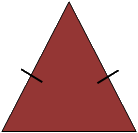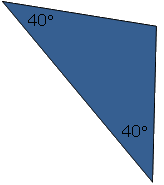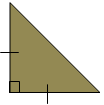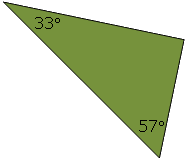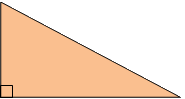Properties of Geometric Figures Activity

Drag each triangle and description from the bottom into the correct section on the Venn Diagram.

Right Triangles Isosceles Triangles
A triangle with a 30° angle and a 60° angle
A triangle with two 45° angles
A triangle with two 60° angles